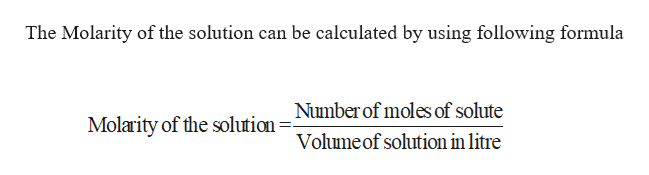# On analysis, an equilibrium mixture for the reaction 2H2S(g) 2H2(g) + S2(g) was found to contain 1.0 mol H2S, 4.0 mol H2, and 0.80 mol S2 in a 4.0 L vessel.  What would the Equilibrium Contant, Kc, be in this equation?

Question
31 views

On analysis, an equilibrium mixture for the reaction 2H2S(g) 2H2(g) + S2(g) was found to contain 1.0 mol H2S, 4.0 mol H2, and 0.80 mol S2 in a 4.0 L vessel.  What would the Equilibrium Contant, Kc, be in this equation?

check_circle

Step 1

Given,

H2S = 1 mol

H2 = 4 mol

S2 = 0.8 mol

In 4.0 L ...help_outlineImage TranscriptioncloseThe Molarity of the solution can be calculated by using following formula Number of moles of solute Molarity of the solution Volume of solution in litre fullscreen

### Want to see the full answer?

See Solution

#### Want to see this answer and more?

Solutions are written by subject experts who are available 24/7. Questions are typically answered within 1 hour.*

See Solution
*Response times may vary by subject and question.
Tagged in

### Chemistry